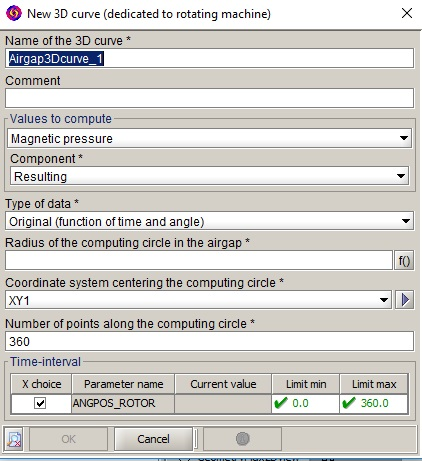# 3D curves dedicated to rotating machines

## General information

The 3D curve dedicated to rotating machine allows computing a formula values along a computing circle positioned around a rotating machine rotation axis. Those values are collected for a set of time steps (feature available only in Transient Magnetic application). Once collected, the values are represented in a 3D curve having in abscises the time and the angular position along the computing circle. A specific option allows eventually to compute those values in 2D Fourier domain depending on the motor-harmonic and the spatial order.

## Creation box options

The creation box of the 3D curve dedicated to rotating machine is presented above:• Values to compute
• Magnetic pressure, allows to compute the magnetic pressure module or one of its components: normal (or radial) component or tangential component. The magnetic pressure at the computation points is obtained by the following formula:

$Pmag=‖n.\frac{{\left(B.n\right)}^{2}-{\left(B.t\right)}^{2}}{2{\mu }_{0}}+t.\frac{\left(B.n\right).\left(B.t\right)}{{\mu }_{0}}‖$

where the first and second terms represent, respectively, the normal and tangential component of the magnetic pressure.
• User formula, allows to compute the values of a user defined spatial formula. This formula should be real scalar.
• Type of data
• Original, returns the values in the time/angular position domain.
• Double FFT, returns the values in the frequency/spatial order domain. Those values are obtained by applying a 2D Fourier transform. The frequencies are normalized by the rotation speed to be expressed in terms of motor-hamonics. .
• Definition of the computing circle
• Radius of the computing circle in the airgap
• Z position of the computing circle in the airgap
• Coordinate system centering the computing circle, allows to adjust the position and the orientation of the computing circle. The circle center is positioned at the coordinate system origin. The circle is plot in the Oxy plane of the coordinate system. The radius dimension unit corresponds to the coordinate system dimension unit. Generally, this coordinate system Oz axis will be aligned with the machine rotation axis.
• Number of points along the computing circle
• Time interval
• Interval, allows to select a set of time (or position) steps. If the data type Double FFT is selected, the interval must represent one complete rotor revolution around its axis to ensure the periodicity of the time evolution.

## 3D curve examples

This 3D curve represents the magnetic pressure evolution along a circle positioned in a rotating machine airgap. This evolution is represented depending on the time and the angular position. In this example, the selected time interval covers one rotating period of the rotor around its axis. This 3D curve represents the magnetic pressure evolution along a circle positioned in a rotating machine airgap. This evolution is represented depending on the motor harmonic and the spatial order. This curve corresponds to the transformation of the previous curve in 2D Fourier domain.